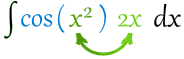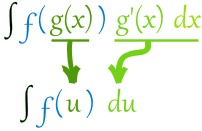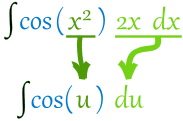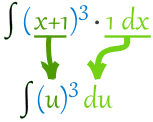# Integration by Substitution

"Integration by Substitution" (also called "u-Substitution" or "The Reverse Chain Rule") is a method to find an integral, but only when it can be set up in a special way.

The first and most vital step is to be able to write our integral in this form:Note that we have g(x) and its derivative g'(x)

Like in this example:Here f=cos, and we have g=x2 and its derivative 2x
This integral is good to go!

When our integral is set up like that, we can do this substitution:Then we can integrate f(u), and finish by putting g(x) back as u.

Like this:

### Example: ∫cos(x2) 2x dx

We know (from above) that it is in the right form to do the substitution:Now integrate:

cos(u) du = sin(u) + C

And finally put u=x2 back again:

sin(x2) + C

So cos(x2) 2x dx = sin(x2) + C

That worked out really nicely! (Well, I knew it would.)

But this method only works on some integrals of course, and it may need rearranging:

### Example: ∫cos(x2) 6x dx

Oh no! It is 6x, not 2x like before. Our perfect setup is gone.

Never fear! Just rearrange the integral like this:

cos(x2) 6x dx = 3cos(x2) 2x dx

(We can pull constant multipliers outside the integration, see Rules of Integration.)

3cos(u) du = 3 sin(u) + C

Now put u=x2 back again:

3 sin(x2) + C

Done!

Now let's try a slightly harder example:

### Example: ∫x/(x2+1) dx

Let me see ... the derivative of x2+1 is 2x ... so how about we rearrange it like this:

x/(x2+1) dx = ½2x/(x2+1) dx

Then we have:Then integrate:

½1/u du = ½ ln(u) + C

Now put u=x2+1 back again:

½ ln(x2+1) + C

### Example: ∫(x+1)3 dx

Let me see ... the derivative of x+1 is ... well it is simply 1.

So we can have this:

(x+1)3 dx = (x+1)3 · 1 dx

Then we have:Then integrate:

u3 du = (u4)/4 + C

Now put u=x+1 back again:

(x+1)4 /4 + C

We can take that idea further like this:

### Example: ∫(5x+2)7 dx

If it was in THIS form we could do it:

(5x+2)7 5 dx

So let's make it so by doing this:

15 (5x+2)7 5 dx

The 15 and 5 cancel out so all is fine.

And now we can have u=5x+2And then integrate:

15 u7 du = 15 u88 + C

Now put u=5x+2 back again, and simplify:

(5x+2)840 + C

Now get some practice, OK?

## In Summary

When we can put an integral in this form:Then we can make u=g(x) and integrate f(u) du
And finish up by re-inserting g(x) where u is.